# Java: Method Overloading

In this article, we will understand Java overloading concepts

Java overloading

• If any class in Java contains multiple methods with exactly same name but with different input parameter lists then it is known as method overloading in Java
• In other words, if 2 or more methods in Java class have same name with different input parameters then it is called as method overloading in Java

Below are the ways to overload methods in Java, by changing

1. Number of input parameters
2. Data-type of input parameters
3. Order of input parameters, if they are of different data-types

Note: return type is not valid to consider for overloading concepts

Advantages of using method overloading in Java

• Maintains consistency with method naming for similar type of tasks
• Increases the readability of the program

Java method signature:

• Instance method (non-static)
• Static method (class method)

Instance method (non-static):

```public int add(int number1, int number2) throws Exception {

return summation;
}
```

Diagrammatic representation of Instance method

Static method (class method):

```public static int add(int number1, int number2) throws Exception {

return summation;
}
```

Diagrammatic representation of Static method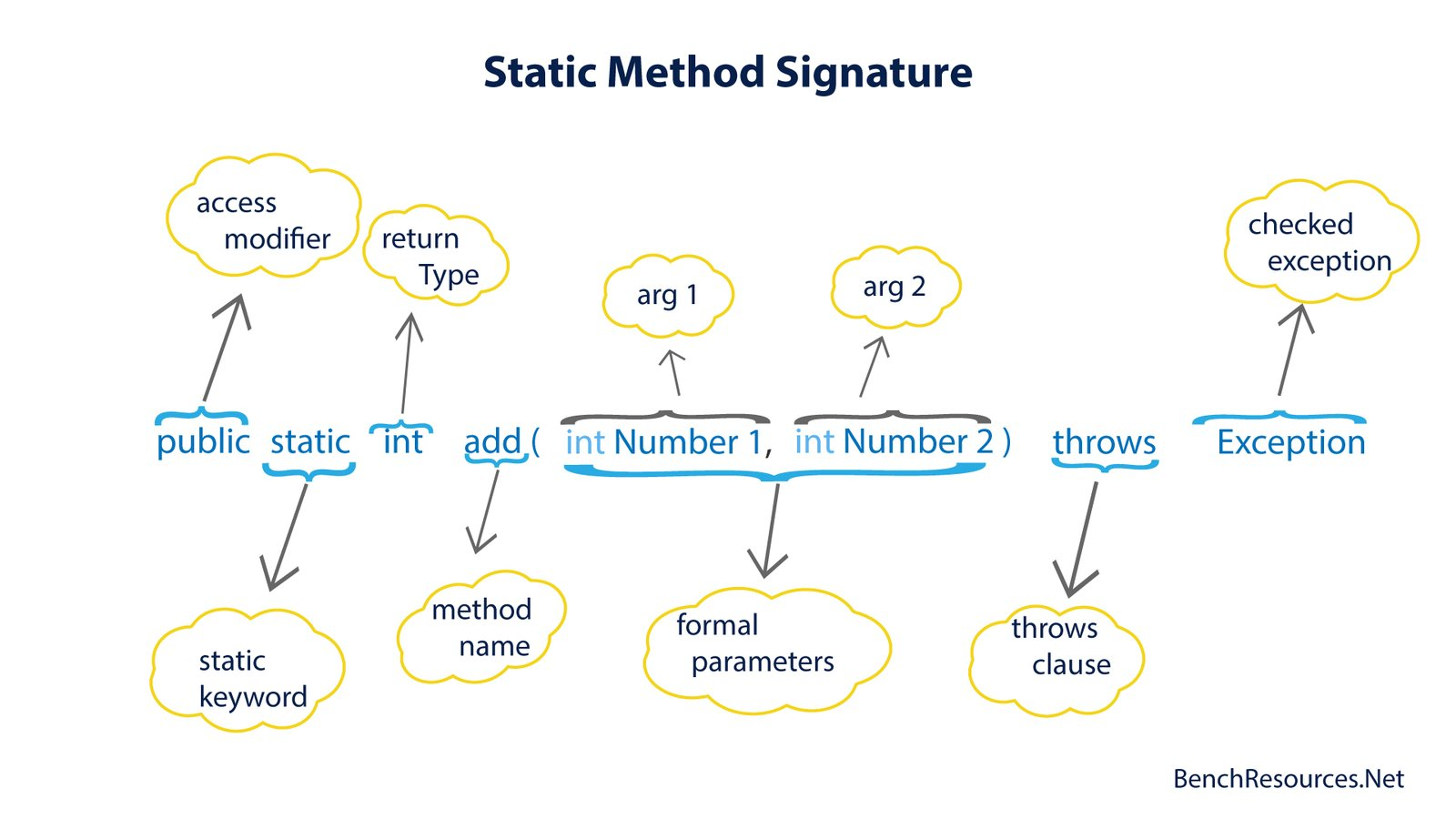Things to remember while overloading (w.r.t method signature)

Compiler checks 3 things when we overload methods

1. method name (should be same)
2. number of input parameters and
3. data-type of input parameters

Other things related to Method Overloading :

• Method name has to be same and combination of number of input parameters & their data-type has to be different for successful compilation
• Or else compiler throws duplicate method error
• Error: Duplicate method method_Name(input parameters) in type ClassName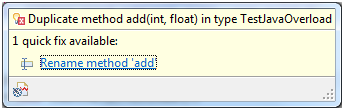• Note: If two method names are different, then it is simply two different methods in a class

Example 1: Method overloading based on number of input parameters

In this example, number of input parameters to overloaded ‘add()’ method is different

• First add() method takes two integer parameters for summation
• whereas second add() method takes two floating numbers for summation and while invoking from main() method we are specifically suffixing ‘f’ for this method call
• third add() method takes three integer parameters for summation of three numbers

TestJavaOverload.java

```package in.bench.resources.java.overload;

public class TestJavaOverload {

void add(int num1, int num2) {
System.out.println("The summation of 2 integer numbers : " + (num1 + num2));
}

void add(float num1, float num2) {
System.out.println("The summation of 2 floating numbers : " + (num1 + num2));
}

void add(int num1, int num2, int num3) {
System.out.println("The summation of 3 integer numbers : " + (num1 + num2 + num3));
}

public static void main(String args[]) {

TestJavaOverload t1 = new TestJavaOverload();
t1.add(12, 16); // method call to 2 integer input parameters list
t1.add(5f, 9f); // method call to 2 floating input parameters list
t1.add(20, 10, 40); // method call to 3 integer input parameters list
}
}
```

Output:

```The summation of 2 integer numbers : 28
The summation of 2 floating numbers : 14.0
The summation of 3 integer numbers : 70
```

Example 2: Method overloading based on data-type or order of input parameters

In this example, data-type or order of input parameters to overloaded ‘add()’ method is different

• Number of input parameters to first & second add() method is two, but they are overloaded based on data-type or say by their order
• First add() method takes two input parameter with 1st being int and 2nd is float
• Whereas next add() method takes same number of input parameters but their order or data-types of input parameter are different i.e.; 1st is float and 2nd is int
• Also, invocations of these two methods are also different. We suffix ‘f’ for float argument for correct and successful invocation

TestJavaOverload.java

```package in.bench.resources.java.overload;

public class TestJavaOverload {

void add(int num1, float num2) {
System.out.println("The summation of 2 numbers : " + (num1 + num2));
}

void add(float num1, int num2) {
System.out.println("Static &amp;gt;&amp;gt; The summation of 2 numbers : " + (num1 + num2));
}

void add(double num1, float num2, long num3) {
System.out.println("The summation of 3 numbers : " + (num1 + num2 + num3));
}

void add(int num1, float num2, double num3) {
System.out.println("The summation of 3 numbers : " + (num1 + num2 + num3));
}

public static void main(String args[]) {

TestJavaOverload t1 = new TestJavaOverload();
t1.add(12, 16f); 		// method call to 2 input parameters list (different data type or order)
t1.add(5f, 9); 			// method call to 2 input parameters list (different data type or order)
t1.add(20d, 10f, 40l); 	// method call to 3 input parameters list (different data type or order)
t1.add(10, 20f, 30d); 	// method call to 3 input parameters list (different data type or order)
}
}
```

Output:

```The summation of 2 numbers : 28.0
The summation of 2 numbers : 14.0
The summation of 3 numbers : 70.0
The summation of 3 numbers : 60.0
```

Implicit type conversion or Type promotion :

• Data-type of input parameter are implicitly promoted for method invocation in case of no matching overloaded methods are available
• In this example, 2nd argument of first method call t1.add(12, 16) is implicitly type promoted to float from int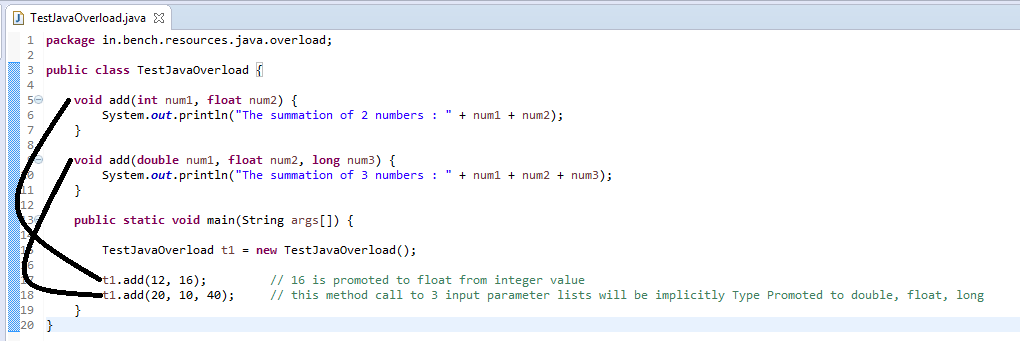Output:

```The summation of 2 numbers : 28.0
The summation of 3 numbers : 70.0
```

Possible type promotion matrix

 Data-type Possible data-type promotion byte short, int, long, float, double short int, long, float, double int long, float, double long float, double float double double – char int, long, float, double

Why method overloading required in Java ?

• Suppose, if we want perform similar kind of tasks and their operation differ only by number of parameters or their data-types or both then method overloading is the best concept to apply
• Example: java.lang.String class from java.lang package contains 9 overloaded ‘valueOf()’ methods with different number of input parameters or their data-types
• In String class, pass a data-type of boolean, char, char array, double, int, long, Object as input parameter to overloaded ‘valueOf()’ method –> it returns String object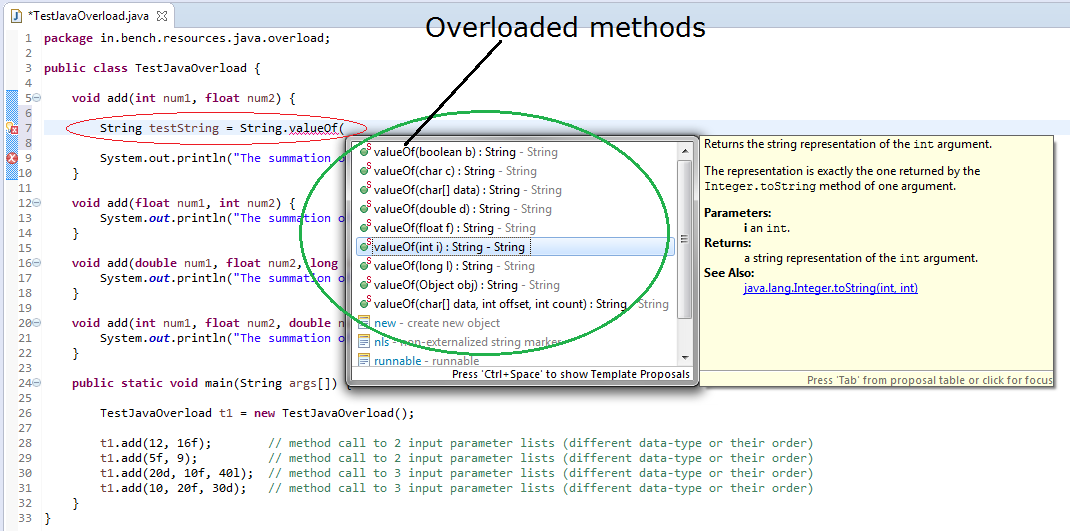Why it is not possible to overload methods based on the return type ?

• Reason is type ambiguity
• First of all, we cannot have two same methods with exactly same input parameters. In this case, compiler throws error as shown in the below screen capture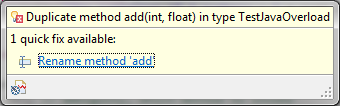• It is a compile-time error, as it is resolved during compile-time
• Also, it is very difficult for JVM to understand as to which version of add(int, float) method to call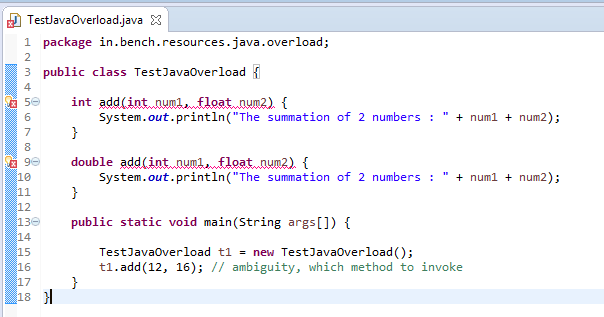In above examples, it is quite evident that compiler checks three things for method overloading

• Method name
• Number of input parameter
• Data-type or order of input parameters

And if it exactly same even when return type is of different, compiler throws error for “duplicate methods” and it also arises type ambiguity situation as to which version of overloaded method needs to be invoked.

So, return-type is not considered for method overloading in Java

Read also:

References:

Happy Coding !!
Happy Learning !!